## Two balls have masses 18 kg and 47 kg. The 18 kg ball has an initial velocity of 76 m/s (to the right) along a line joining the two balls an

Question

Two balls have masses 18 kg and 47 kg. The 18 kg ball has an initial velocity of 76 m/s (to the right) along a line joining the two balls and the 47 kg ball is at rest. They make a head-on elastic collision with each other. What is the final velocity of the 18 kg ball?

in progress 0
5 months 2021-08-15T00:27:23+00:00 1 Answers 17 views 0

The final velocity of 18 kg ball is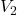= 42.09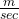Explanation:

Mass of first ball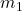= 18 kg

Mass of second ball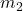= 47 kg

Initial velocity of 18 kg ball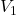= 76Initial velocity of 47 kg ball = 0

Final velocity of 18 kg ball= ??

Final velocity of 18 kg ball  is given by the formula=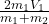Put all the values in above formula we get= 2 × 18 ×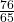= 42.09Thus, the final velocity of 18 kg ball is= 42.09